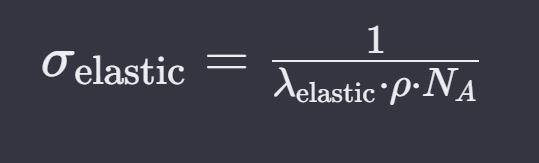# Measurement of cross section

Dear experts, i am running simple experiment in FLUKA…in the basic experiment…I want to measure the cross sections of radionuclides after spallation of uranium with 100Mev proton…Is it sufficient to use simple Resnuclie card…how to get values in mb…please put some light.

you can use a RESNUCLEi scoring, to get the isotope production per primary particle. Then you need to normalize the result by the p-U cross section (that can be retrieved from the inelastic scattering length in the output material table) and by the reaction probability (number of proton stars in the output too, assuming they take place in the target only)

You need to model a thin target (not to change the proton energy through it and be affected by re-interactions) and you may need to use biasing the proton reaction probability using LAM-BIAS.

Cheers,
Daviddear Sir, do i need to use these formulas to normalize the isotopes production, will it give the cross section in barn? I try to calculate through this but, my normalization factor comes around 1.09E+15cm-2, and i divide it by isotopes production number, suppose if it is 3.44E-04, the overall results comes out to be 3.15E-19cm2, and if i want to get in barn, the result will be 315000 barn…which seem error…please advise

Your normalization factor is composed of two numbers:

sigma [mb] = A [g/mol] / ( lambda [cm] * rho [g/cm^3] * N_A [mol^-1] ) * 10^27
where A is the molar mass, rho is the material density, and N_A is the Avogadro number.

Number of stars generated per beam particle: